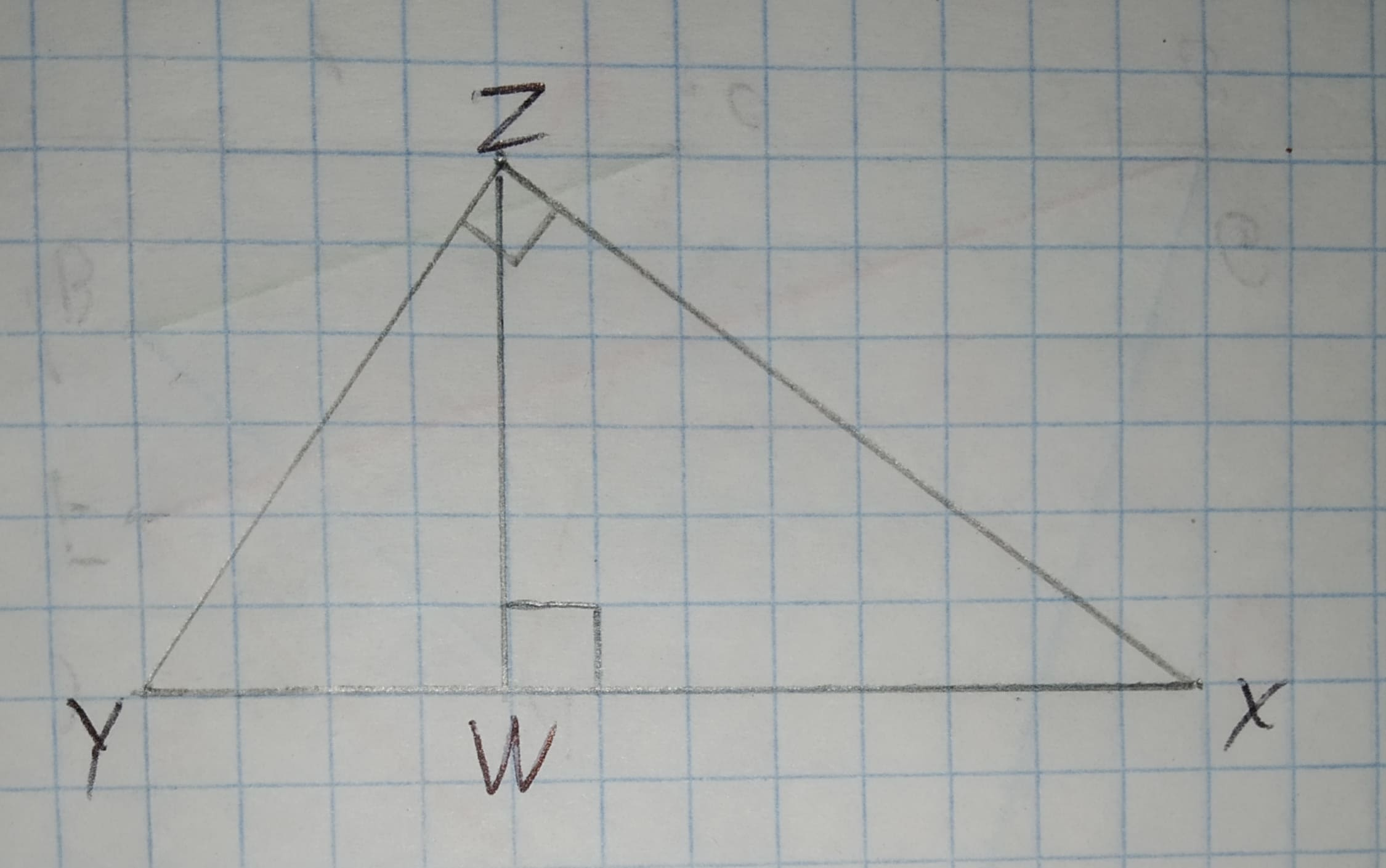# To write three similarity statements about the triangles and the reason that they are similar.Given that12210203151.jpgopatovaL 2021-01-07 Answered

To write three similarity statements about the triangles and the reason that they are similar.
Given thatYou can still ask an expert for help

• Questions are typically answered in as fast as 30 minutes

Solve your problem for the price of one coffee

• Math expert for every subject
• Pay only if we can solve itClara Reese
The triangle XYZ and the triangles XWZ and YWZ are right triangles.
Since,
$\mathrm{\angle }ZWX=\mathrm{\angle }YZX=\mathrm{\angle }YZW={90}^{\circ }$
Also, midpoint of line XY divide the base proportionally.
Therefore, the sides are also proportional.
Therefore, by the rules of similarity, the triangles are proportional.